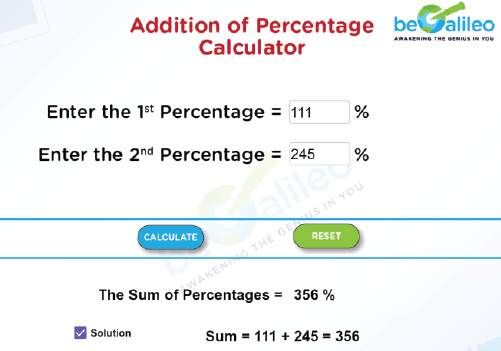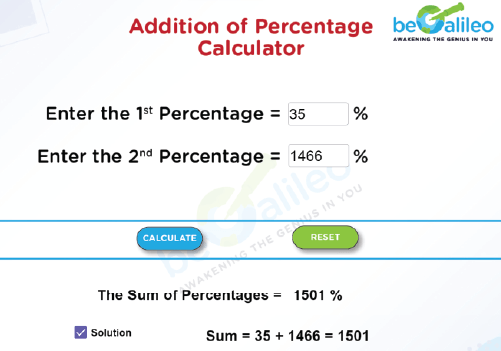>### Master Math with 1:1 Online Classes. Learn from Experts!Addition of Percentage Calculator is an online calculator tool which helps you to add two percentages in no time. This calculator will calculate the total percentage when we enter the percentages to be added.

A percentage is a proportion of a whole number, expressed as a value out of a hundred. It is represented by the percentage symbol “%”. The formula to calculate percentage is given by

Percentage, % = (\frac{Value}{Total Value}) x 100

Percentages are important for understanding the financial conditions of routine life. They are frequently used to calculate discounts, taxes, interest rates, average player values in sports, rankings, surveys, weather reports, etc.

## How to use the Addition of Percentage Calculator?

Step 1: Type the percentage values whose sum must be found into the text boxes.

Step 2: Click “CALCULATE”, the sum will be displayed on the screen.

Step 3: Select the Solution checkbox to view the detailed calculation.

Step 4: Click “RESET”. The previous calculations will be erased so that the new values can be entered.

## How to find the sum of the percentages?

We should add the percentages given to find their sum.

Let A and B be the two percentages given. Then the formula used to find the Sum of A and B,

Sum of A and B = A + B

Finally, the answer should be denoted by a ‘ % ’ symbol.

## Solved Examples

### Q1. Find the sum of the percentages 111 and 245.

Solution :
Sum = (111 + 245)%
= 356 %
The sum of 111 % and 245 % is 356 %.### Q2. Find the sum of 35 % and 1466 %.

Solution :
Sum = (35 + 1466)%
= 1501 %
The sum of 35 % and 1466 % is 1501 %.